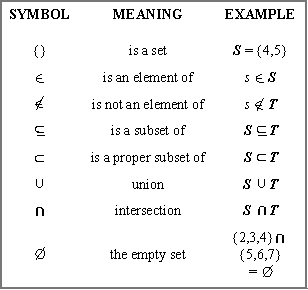# Do You Know Sets?

10 Questions | Total Attempts: 112SettingsA set is a well-defined collection of distinct objects. Set is well known topic in mathematics. Lovers of mathematics show know a lot about it. I'm challenging you take this quiz to test your knowledge on the topic?

Related Topics
• 1.
Which of the following is known as the objects that makes up sets?
• A.

Elements

• B.

Member

• C.

All of the above

• D.

None of the above

• 2.
Sets are conventionally denoted with capital letters.
• A.

True

• B.

False

• C.

Sometimes

• D.

Maybe

• 3.
How many ways are used in describing or specifying the members of a set?
• A.

1

• B.

2

• C.

3

• D.

4

• 4.
Which of the following is a way of describing elements in a set?
• A.

Intensional

• B.

Extensional

• C.

Inferior

• D.

Interior

• 5.
Which of the following is a way of describing elements in a set?
• A.

Interior

• B.

Extensional

• C.

Exterior

• D.

None of the above

• 6.
Which of the following means "B is a set and x is one of the objects of B"?
• A.

x ⊂ B

• B.

x∉ B

• C.

x ∈ B

• D.

None of the above

• 7.
Which of the following means y is not a member of B?
• A.

Y∈ B

• B.

Y ∉ B

• C.

Y ⊆ B

• D.

None of the above

• 8.
"If every member of set A is also a member of set B, then A is said to be a subset of B".Which of the following means the above statement?
• A.

A ∉ B

• B.

A ∈ B

• C.

A ⊆ B

• D.

Y ∉ B

• 9.
Which of the following is an operation in set?
• A.

Union

• B.

Join

• C.

Marry

• D.

Knitted

• 10.
Which of the following is an operation in set?
• A.

Intersection

• B.

Complements

• C.

None of the above

• D.

All of the above